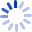# A Beginner's Guide to Structural Equation Modeling

This book provides the reader with a review of correlation and covariance among variables, followed by multiple regression and path analysis techniques to better understand the building blocks of structural equation modelling. The concepts behind measurement models are introduced to illustrate how measurement error impacts statistical analyses, and structural models are presented that indicate how latent variable relationships can be established. Examples are included throughout to make the concepts clear to the reader. The ...

##### Cash for Textbooks